# Differential Amplifier (Subtractor)

## Working principle

A differential op-amp amplifies the difference between two input voltages (Vin1 and Vin2).

### Formula derivation

If Vin2 = 0,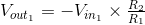If Vin1 = 0,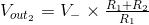Given that: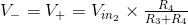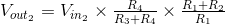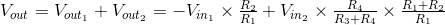Experiments

• Equate R1 to R3, and R2 to R4 to transform this circuit into a voltage subtractor with the output (R2/R1)*(Vin2-Vin1).
• Equate all resistor values. What is the output in terms of the input voltages now?